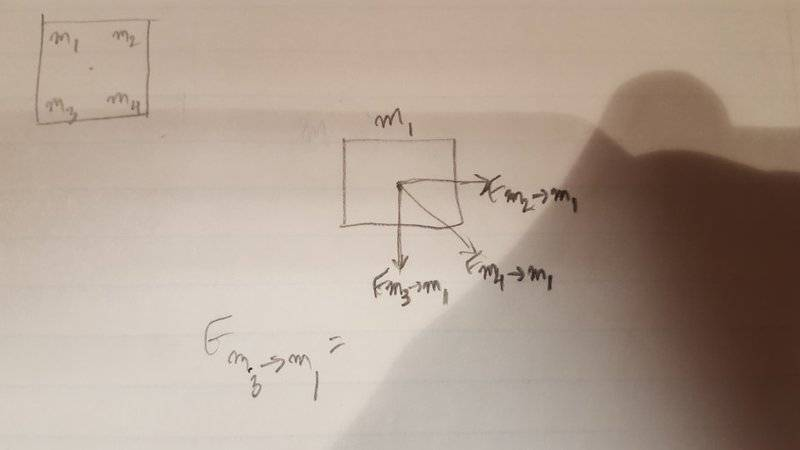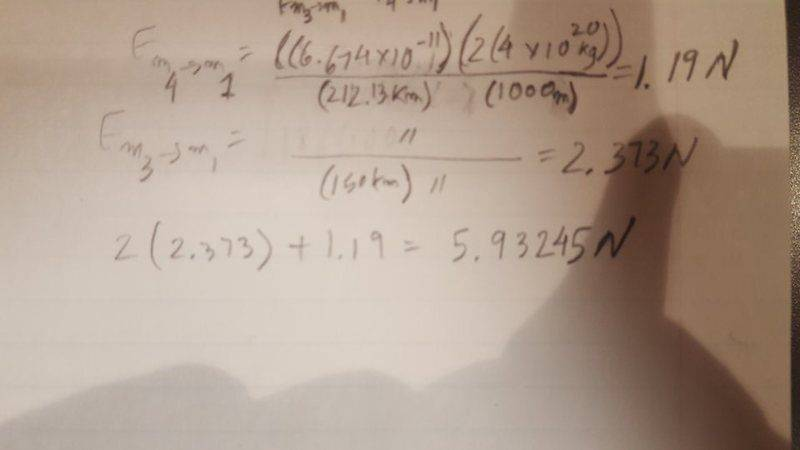# College physics problem -- Find gravitational acceleration

## Homework Statement

Here's the question:

As space colonization expands, it's important to build new stations from local materials instead of bringing everything from Earth. Your latest task (besides asking for a raise) is to check the long-term stability of a proposed configuration of asteroids for a deep-space station, far from any stars. The schematic you receive has four 4 x 10^20 kg asteroids configured so that each is at the corner of a square with 150 km sides. The asteroids will gravitationally attract each other, but the designer claims it will be stable for hundreds of years. To check, you want to calculate the acceleration of one of the asteroids in the proposed configuration. Make sure you give both the magnitude and the direction of the acceleration.

Hint: Consider just one of the asteroids and analyze the forces it will feel from the other three.

Note: Far away from Earth, we can't use a constant gravitational force Fg = mg. Instead, we need to use a more general expression: Fg = (Gm1m2)/r^2 , where m1 and m2 are two objects' masses, r is the distance between them, and G is a constant, G = (6.674 x 10^-11)N*m^2/kg^2

## Homework Equations

Fg = (G*m1m2)/r^2

## The Attempt at a Solution

All I have done is start the free body diagram of one of the asteroids:
Can I find the resultant of F m3->m1 and F m2->m1 and add that to F m4->m1 to find the total Fg towards the center?

I'm stuck. Any tips on getting started?
(752 + 752) = 106.07km
asteroid has to travel 106.07km to get to the center.

Last edited:
•berkeman

berkeman
Mentor

## Homework Statement

Here's the question:

As space colonization expands, it's important to build new stations from local materials instead of bringing everything from Earth. Your latest task (besides asking for a raise) is to check the long-term stability of a proposed configuration of asteroids for a deep-space station, far from any stars. The schematic you receive has four 4 x 10^20 kg asteroids configured so that each is at the corner of a square with 150 km sides. The asteroids will gravitationally attract each other, but the designer claims it will be stable for hundreds of years. To check, you want to calculate the acceleration of one of the asteroids in the proposed configuration. Make sure you give both the magnitude and the direction of the acceleration.

Hint: Consider just one of the asteroids and analyze the forces it will feel from the other three.

Note: Far away from Earth, we can't use a constant gravitational force Fg = mg. Instead, we need to use a more general expression: Fg = (Gm1m2)/r^2 , where m1 and m2 are two objects' masses, r is the distance between them, and G is a constant, G = (6.674 x 10^-11)N*m^2/kg^2

## Homework Equations

Fg = (G*m1m2)/r^2

## The Attempt at a Solution

All I have done is start the free body diagram of one of the asteroids. I'm stuck. Any tips on getting started?
(752 + 752) = 106.07km
Can you post a (clear) picture of your FBD? You can use the UPLOAD button to upload a *.JPG picture. You should be showing the 4 masses at the corners of the square, with three force vectors shown on one of the four masses... Can you write the sum of the 3 vector forces on that one mass?

Can you post a (clear) picture of your FBD? You can use the UPLOAD button to upload a *.JPG picture. You should be showing the 4 masses at the corners of the square, with three force vectors shown on one of the four masses... Can you write the sum of the 3 vector forces on that one mass?

berkeman
Mentor
The UPLOAD button is in the lower right part of the Reply dialog box. At least it is on PCs and Laptops, I'm not sure if it works with phones and tablets.

Your FBD looks fine. So now just write the 3 vector forces and sum them up vectorially. The force depends on the masses and the separation distances, as you listed in your OP.The UPLOAD button is in the lower right part of the Reply dialog box. At least it is on PCs and Laptops, I'm not sure if it works with phones and tablets.

Your FBD looks fine. So now just write the 3 vector forces and sum them up vectorially. The force depends on the masses and the separation distances, as you listed in your OP.I calculated F m3->m1(2372978N) + F m2->m1(2372978N) + F m4->m1(1186400N) and got 5932356N
Is that correct?
(EDIT: this is wrong)

Last edited:
berkeman
Mentor
I calculated F m3->m1(2372978N) + F m2->m1(2372978N) + F m4->m1(1186400N) and got 5932356N
Is that correct?

Beats me. Can you show how you summed the x & y components of each force to get the overall x & y force components? And then how that gives you the overall magnitude and direction of the net force?

berkeman
Mentor
Make sure you give both the magnitude and the direction of the acceleration.
BTW, the answer they are looking for is the final resultant acceleration, not just the force.Beats me. Can you show how you summed the x & y components of each force to get the overall x & y force components? And then how that gives you the overall magnitude and direction of the net force?So 5.9N is the total force m4 and m1. And then I plan on using f = ma.
use (5.9N = (4 * 10^20) * a) to find acceleration?
btw, I found 212.13km as the distance between m4 and m1 by using sqrt(150^2+150^2)

berkeman
Mentor
View attachment 96091
So 5.9N is the total force m4 and m1. And then I plan on using f = ma.
use (5.9N = (4 * 10^20) * a) to find acceleration?
btw, I found 212.13km as the distance between m4 and m1 by using sqrt(150^2+150^2)
It's a little hard to read what you are doing, but it sounds like you are taking the right approach. I'd draw a new version of the 4-mass square, and label the 3 forces acting on m1 as vectors aiming at the respective masses. Then show separate sums for the x & y components, using an x-y coordinate system centered on m1. Then it should be easy to add up the vector components and keep going.It's a little hard to read what you are doing, but it sounds like you are taking the right approach. I'd draw a new version of the 4-mass square, and label the 3 forces acting on m1 as vectors aiming at the respective masses. Then show separate sums for the x & y components, using an x-y coordinate system centered on m1. Then it should be easy to add up the vector components and keep going.calculated the resultant of F m2->m1 and F m3->m1 = 3.356N
3.356 + 1.19(F m4->m1) = 4.546N
a = 4.456N/(4 * 10^20kg) = 1.114*10^-20 m/s^2
Since you're the only one replying, Can you please do the math to make sure the acceleration is correct?

berkeman
Mentor
calculated the resultant of F m2->m1 and F m3->m1 = 3.356N
3.356 + 1.19(F m4->m1) = 4.546N
a = 4.456N/(4 * 10^20kg) = 1.114*10^-20 m/s^2
Since you're the only one replying, Can you please do the math to make sure the acceleration is correct?
But the three force vectors point in different directions. I'm not understanding how you handled that part...

SteamKing
Staff Emeritus